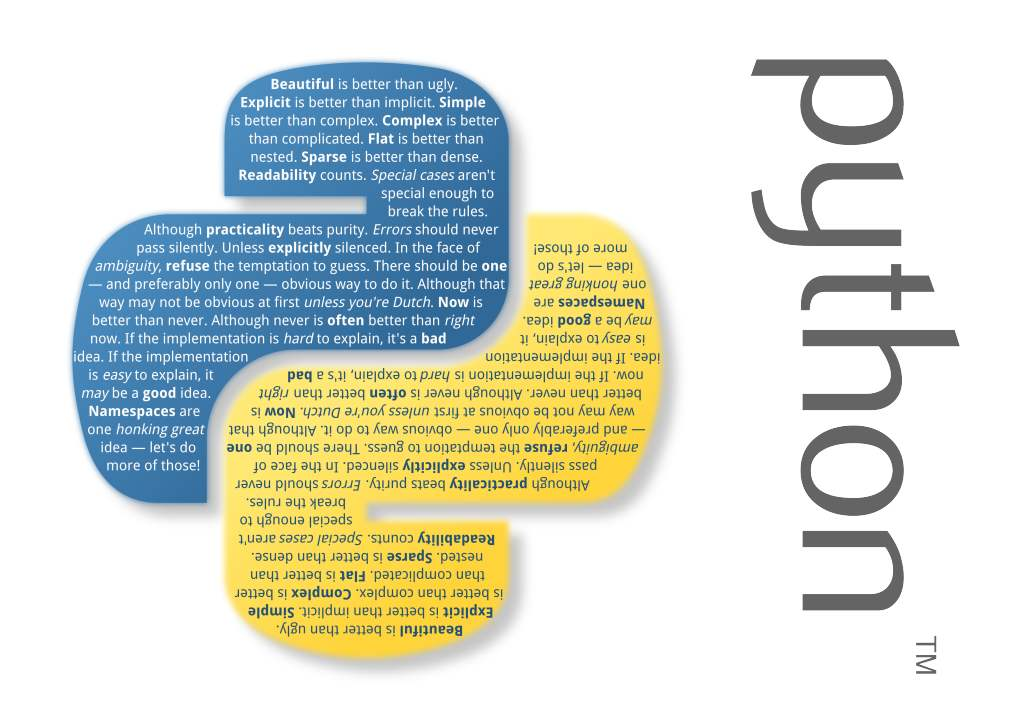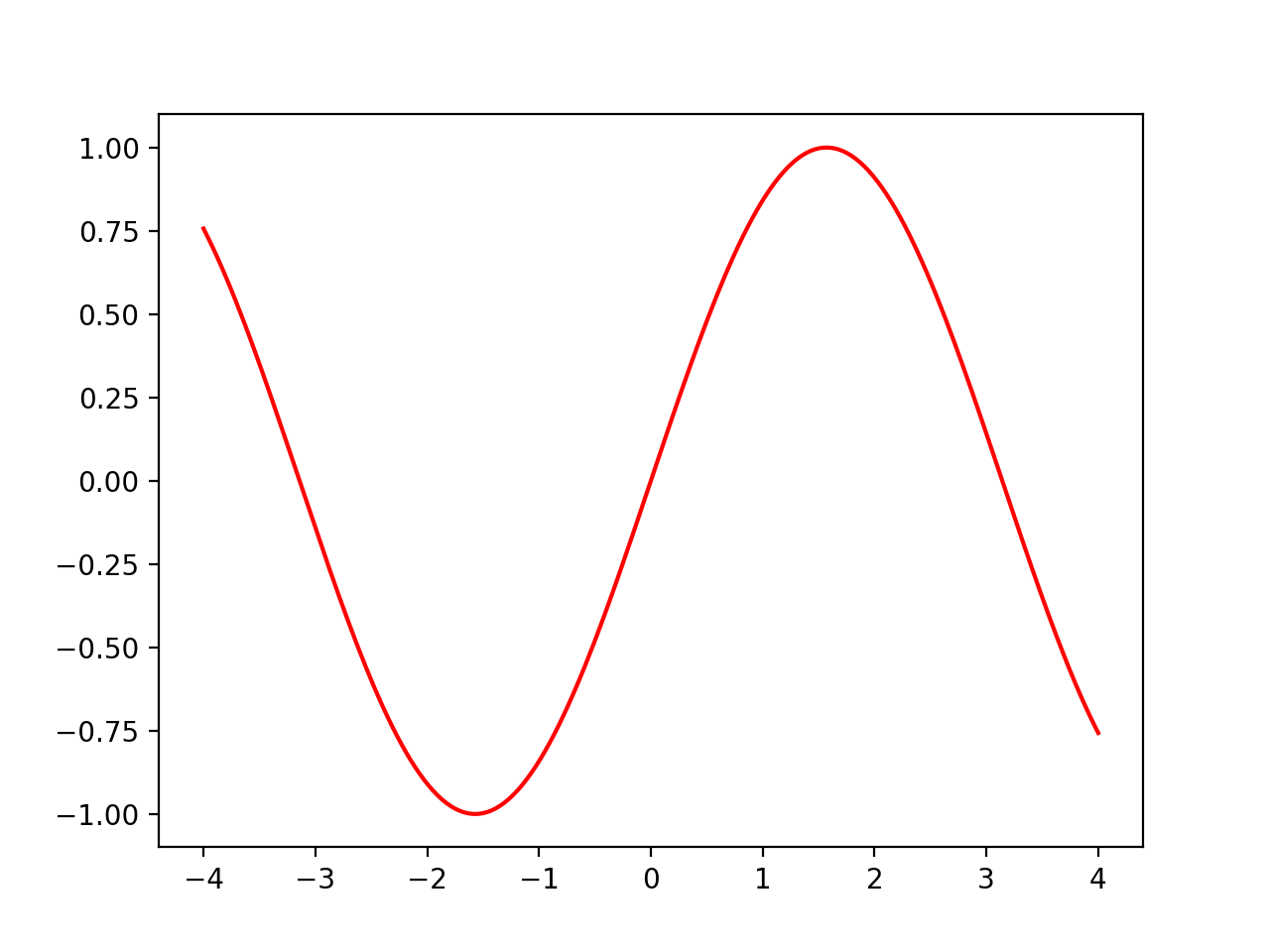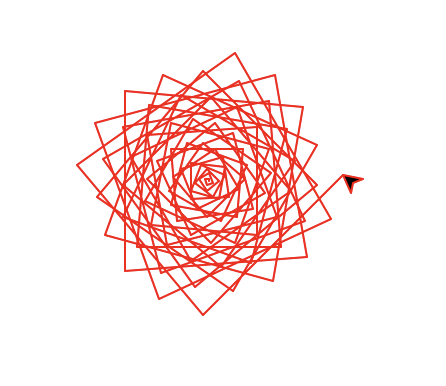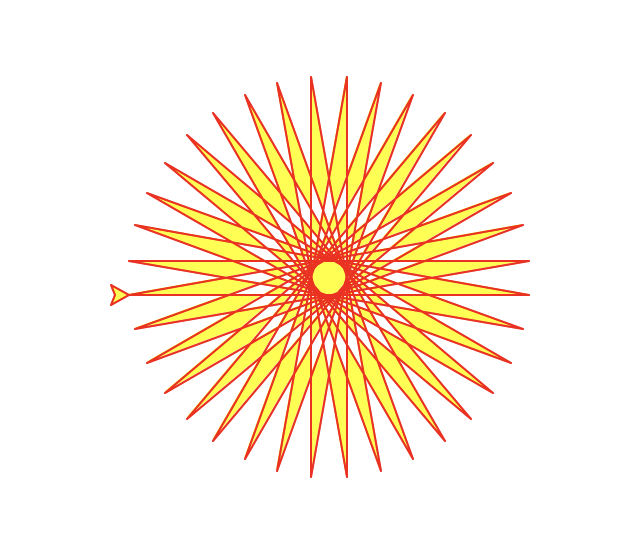# 从零开始学习PYTHON3讲义（十二）（内容需要，本讲使用了大量在线公式，如果因为转帖网站不支持公式无法显示的情况，欢迎访问原始博客。）

### 《从零开始PYTHON3》第十二讲

#### Python科学绘图

#首先使用管理员模式执行cmd命令行，随后在命令行执行：
pip install matplotlib  #某些系统需要使用pip3


$$y = f(x)$$

#绘制正弦曲线

#引入数值计算库,改为短名称
import numpy as np
#引入绘图库，改为短名称
import matplotlib.pyplot as plt

#生成一个由-4到4、均分为200个元素的列表
x = np.linspace(-4, 4, 200)
#计算当x取值范围-4至4时所有的sin函数解
f = np.sin(x)

#绘制
plt.plot(x, f, 'red')

#将绘制好的图显示出来
plt.show()


• np.linspace函数我们在讲数值计算的时候学过，是生成一个200个元素的列表，这个列表是numpy库的列表类型，跟python内置的列表是基本兼容的，但并不是同一种类型。这200个元素均分了从-4到+4的值范围，包含了-4/+4本身。
• np.sin(x)，看起来跟内置的math.sin(x)很像，事实上当x是一个数字变量的时候，两者完全相同。但在这里，x是一个列表，包含200个元素。那两者就完全不同了。内置的math.sin一次调用只能处理一个数字。np.sin是一次处理整个数组。因此调用完成后，结果f中是包含了200个值，每个相同下标的值，是对应x列表中对应下标值的正弦函数结果值。所以f在这里实际就是y坐标的值。
• 终于看到了plt.plot函数，里面有三个参数，x是x坐标列表，本例中包含了200个元素，f实际是y轴坐标列表，也包含了200个元素，最后的’red’表示使用红色绘制。此函数在绘制这个数组的时候，每两个点之间，默认会使用直线连接上，从而让整体上形成一条平滑的曲线。
• 最后plt.show()是把绘制好的图形显示出来，此语句之后的绘图将被忽略掉，所以请确保这一条语句是所有plt调用的最后一句。干净漂亮的正弦曲线出现了！

Python的学习一定要多动手练习，所以请自己也来来试试绘制过程。比如改变参数范围从-10到+10，比如把200个列表元素改成只有10个，看看是什么效果？

#为某条曲线增加注释文字
plt.text(1.3, 0.9, 'sin(x)',color='b')

#为整个图增加标题
plt.title('y=f(x)', fontsize=16)


$$y=ax+b$$

#绘制直线

#引入数值计算库
import numpy as np
#引入绘图库
import matplotlib.pyplot as plt

#定义一个直线函数
def line(x,a,b):
return a*x+b

#我们用于示例，定义的函数没有计算数组的功能
x1=-4
y1=line(x1,0.2,0)
x2=4
y2=line(x2,0.2,0)

#绘制直线，只需要两个端点
plt.plot([x1,x2],[y1,y2],'r')

#增加注释文字
plt.text(-3.9, -0.8, '0.2*x',color='r')
#为整个图增加标题
plt.title('y=ax+b', fontsize=16)

#将绘制好的图显示出来
plt.show()


#### 海龟绘图

#海龟绘图演示

#引入海龟绘图库
import turtle

#建立一支笔（一只海龟）
t = turtle.Pen()
for x in range(100):
#向前走x步
t.forward(x)
#左转90度
t.left(90)
turtle.done()


#海龟绘图演示

#引入海龟绘图库
import turtle

#建立一支笔（一只海龟）
t = turtle.Pen()
t.pencolor('red')
for x in range(100):
#向前走x步
t.forward(x)
#左转95度
t.left(95)
turtle.done()除了设置画笔的颜色，还可以设置画笔所封闭的区域中的填充颜色，请看这个例子和执行的结果：

#引入绘图库
from turtle import *

#设置笔的颜色和填充颜色
color('red', 'yellow')
#开始填充
begin_fill()
while True:
forward(200)
left(170)
#pos是当前海龟坐标
#abs(pos())表示判断如果
# 海龟回到原点附近则停止
if abs(pos()) < 1:
break
#结束填充
end_fill()
done()#### 练习时间

1.请绘制下面函数的图像：
$$y=\sqrt{1-(|x|-1)^2},\arccos(1-|x|)-\pi$$

• y是两个公式，用逗号隔开，表示取值是两个，程序中应当分别计算，得到两组值
• x取值空间建议：-2至2
• 根号函数：numpy.sqrt()，绝对值：numpy.fabs()
• 平方：numpy.square(),同**2的区别，后者只计算一个值，前者计算整个列表
• 其它函数：numpy.arccos,常量π:numpy.pi

2.海龟绘图的练习，其实在第一讲的时候我们练习过一些了，现在学习了这么多，再来试试吧
A.修改前面例程的简单参数，构建有趣的规则几何图形
B.开动脑筋，重新编程，绘制一副更有创意的图形

#### 本讲小结

• 图形、图像是计算机科学中重要的组成部分
• 科技绘图用途广泛，也是理工学习中必须用到的内容
• 海龟绘图简单有趣，能显示绘图过程，适合简单创意性的场合

#### 练习答案

1.课程中的思考题，在自定义函数中，应当使用循环，遍历参数的所有元素，逐个代入数学公式中计算，得到的结果逐个加入已经预先定义好的空列表中，最终返回这个完整的列表。程序代码略。

2.请参考ex1.py程序

3.海龟绘图练习略